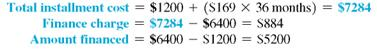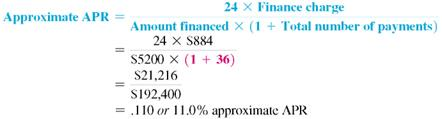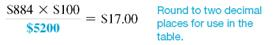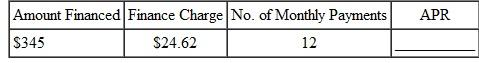# [Solved] Find the Annual Percentage Rate Using the Annual Percentage Rate

Question 80
Essay

## Find the annual percentage rate using the annual percentage rate table. (See Example.) Finding the Annual Percentage Rate In Example, a used car costing \$6400 was financed at \$169 per month for 36 months after a down payment of \$1200. The total finance charge was \$884, and the amount financed was \$5200. Find the annual percentage rate. Finding the Annual Percentage Rate Ed Chamski decides to buy a used car for \$6400. He makes a down payment of \$1200 and monthly payments of \$169 for 36 months. Find the approximate annual percentage rate rounded to the nearest tenth of a percent. SOLUTION Use the steps outlined above.Quick TIP The precise APR can be found using a financial calculator as shown in examples in Appendix C. Use the formula for approximate APR. Replace the finance charge with \$884, the amount financed with \$5200, and the number of payments with 36.The approximate annual percentage rate on this loan is 11%. Example shows how to find the actual APR for this loan. SOLUTION Step 1 Multiply the finance charge by \$100, and divide by the amount financed. Quick TIP When using the annual percentage rate table, select the column with the table number that is closest to the finance charge per \$100 of amount financed.This gives the finance charge per \$100 financed. Step 2 Read down the left column of the annual percentage rate table to the line for 36 months (the actual number of monthly payments). Follow across to the right to find the number closest to \$17.00. Here, find 17.01. Read the number at the top of this column of figures to find the annual percentage rate, 10.50%. In this example, 10.50% is the annual percentage rate that must be disclosed to the buyer of the car. In Example, the formula for the approximate annual percentage rate gave an answer of 11%, which is not accurate enough to meet the requirements of the law. Finding the Annual Percentage Rate Ed Chamski decides to buy a used car for \$6400. He makes a down payment of \$1200 and monthly payments of \$169 for 36 months. Find the approximate annual percentage rate rounded to the nearest tenth of a percent. SOLUTION Use the steps outlined above.Quick TIP The precise APR can be found using a financial calculator as shown in examples in Appendix C. Use the formula for approximate APR. Replace the finance charge with \$884, the amount financed with \$5200, and the number of payments with 36.The approximate annual percentage rate on this loan is 11%. Example shows how to find the actual APR for this loan.10+ million students use Quizplus to study and prepare for their homework, quizzes and exams through 20m+ questions in 300k quizzes.

Explore our library and get Management Homework Help with various study sets and a huge amount of quizzes and questions

3.5K

Study sets

66.3K

Quizzes

5.9M

Questions

Upload material to get free accessInvite a friend and get free accessSubscribe and get an instant access